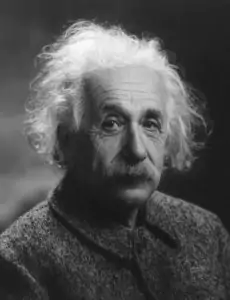Albert Einstein’s most famous contribution to physics, the Theory of Relativity, is known by name around the world, but the majority of people do not know about it in depth. This is because the theory is relatively difficult to grasp. Most people do not even know that the thoery encompases two interralted theories: special relativity and general relativity. Therefore, the following paragraphs will aid people in knowing more about this essential theory of physics.Use the most powerful academic tools to write better with AI, check for plagiarism and detect AI content!

Firstly, we need to understand the difference between the two theories encapsulated in the Theory of Relativity. Special relativity is the first component of the main theory. According to Space.com when explaining special relativity, “Simply put, as an object approaches the speed of light, its mass becomes infinite and it is unable to go any faster than light travels. This cosmic speed limit has been a subject of much discussion in physics, and even in science fiction, as people think about how to travel across vast distances. The theory of special relativity explains how space and time are linked for objects that are moving at a consistent speed in a straight line” (Howell, Elizabeth). This theory is based on two postulates proposed by Einstein in a paper called “On the Electrodynamics of Moving Bodies”:

1) The laws of physics are invariant (i.e., identical) in all inertial systems (i.e., non-accelerating frames of reference).

2) The speed of light in a vacuum is the same for all observers, regardless of the motion of the light source.

The theory of special relativity is the most accurate model of motion when the influence of gravity is barely traceable. It also has many consequences, one of which is time being perceived not as absolute, but rather dependent on a frame of reference and position in space.

Moving on to the theory of general relativity, it is now the modern description of gravitation. It gives us a unified look at gravitation, as it generalizes special relativity and Newton’s law of universal gravitation. According to New Scientist, “it explains that what we perceive as the force of gravity in fact arises from the curvature of space and time. Einstein proposed that objects such as the sun and the Earth change this geometry. In the presence of matter and energy it can evolve, stretch and warp, forming ridges, mountains and valleys that cause bodies moving through it to zigzag and curve” (“Instant Expert: General Relativity”). So, the existence of matter in spacetime influences whatever is around it in the form of gravity. The curvature of spacetime is essentially gravity.

The Theory of Relativity has many implications. First of all, the famous equation E=mc2 means that energy is mass times the speed of light squared. This elegant equation showed that mass and energy are interchangeable (“Today in Science: Albert Einstein and E=Mc2.”). The theory also infers the fact of time dilation, which is “The slowing of the passage of time experienced by objects in motion relative to an observer; measurable only at relativistic speeds” (Boundless). Therefore, if one person is going at a higher velocity than another person for a certain period of time, the person who is experiencing a lower velocity will measure time differently. The person who spent time at a higher velocity will measure time in a shorter manner. In addition, length contraction is implied by the Theory of Relativity. If an object moves at a rate close to the speed of light, or significant fractions of that speed, the object can be observed as being shorter. This is an actual, observable shortness of length. However in its zero-velocity state, its length would return to normal.

Above were just a few implications of the theory. There is so much more to discuss, as one can imagine. However, for the purpose of this essay, let us backtrack and redefine the Theory of Relativity. It is a combination of special relativity (basically, when matter approaches the speed of light, its mass becomes infinite and cannot go faster than the speed of light) and general relativity (gravity is the curvature of spacetime through the presence of matter). This is the most basic breakdown of the theory, but it has changed how we look at classical physics and our physical universe.

## Works Cited

Howell, Elizabeth. “Einstein’s Theory of Special Relativity.” Space.com, Space.com, 30 Mar. 2017, www.space.com/36273-theory-special-relativity.html.

“Instant Expert: General Relativity.” New Scientist, New Scientist, www.newscientist.com/round-up/instant-expert-general-relativity/.

“Today in Science: Albert Einstein and E=Mc2.” EarthSky, earthsky.org/human-world/this-date-in-science-emc2.

Boundless. “Boundless Physics.” Lumen Learning, Lumen, courses.lumenlearning.com/boundless-physics/chapter/implications-of-special-relativity/.